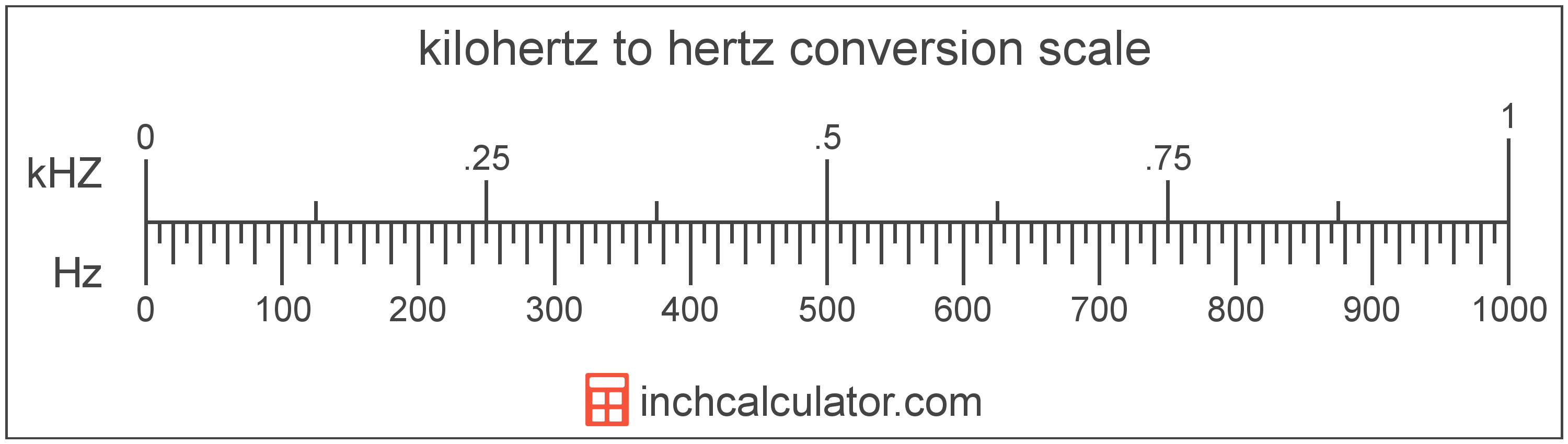# Hertz to Kilohertz Converter

Enter the frequency in hertz below to get the value converted to kilohertz.

Results in Kilohertz:1 Hz = 0.001 kHZ

Do you want to convert kilohertz to hertz?

## How to Convert Hertz to Kilohertz

To convert a measurement in hertz to a measurement in kilohertz, divide the frequency by the following conversion ratio: 1,000 hertz/kilohertz.

Since one kilohertz is equal to 1,000 hertz, you can use this simple formula to convert:

kilohertz = hertz ÷ 1,000

The frequency in kilohertz is equal to the frequency in hertz divided by 1,000.

For example, here's how to convert 5,000 hertz to kilohertz using the formula above.
kilohertz = (5,000 Hz ÷ 1,000) = 5 kHZ## What Is a Hertz?

One hertz is formally defined as the frequency of one cycle per second.

The hertz is the SI derived unit for frequency in the metric system. Hertz can be abbreviated as Hz; for example, 1 hertz can be written as 1 Hz.

Frequency in hertz can be expressed using the formula:

Hz = Cycles / Time in Seconds

## What Is a Kilohertz?

Kilohertz is a measure of frequency equal to one thousand cycles per second.

The kilohertz is a multiple of the hertz, which is the SI derived unit for frequency. In the metric system, "kilo" is the prefix for thousands, or 103. Kilohertz can be abbreviated as kHZ; for example, 1 kilohertz can be written as 1 kHZ.

## Hertz to Kilohertz Conversion Table

Table showing various hertz measurements converted to kilohertz.
Hertz Kilohertz
1 Hz 0.001 kHZ
2 Hz 0.002 kHZ
3 Hz 0.003 kHZ
4 Hz 0.004 kHZ
5 Hz 0.005 kHZ
6 Hz 0.006 kHZ
7 Hz 0.007 kHZ
8 Hz 0.008 kHZ
9 Hz 0.009 kHZ
10 Hz 0.01 kHZ
20 Hz 0.02 kHZ
30 Hz 0.03 kHZ
40 Hz 0.04 kHZ
50 Hz 0.05 kHZ
60 Hz 0.06 kHZ
70 Hz 0.07 kHZ
80 Hz 0.08 kHZ
90 Hz 0.09 kHZ
100 Hz 0.1 kHZ
200 Hz 0.2 kHZ
300 Hz 0.3 kHZ
400 Hz 0.4 kHZ
500 Hz 0.5 kHZ
600 Hz 0.6 kHZ
700 Hz 0.7 kHZ
800 Hz 0.8 kHZ
900 Hz 0.9 kHZ
1,000 Hz 1 kHZ

## References

1. National Institute of Standards and Technology, SI Units - Time, https://www.nist.gov/pml/owm/si-units-time